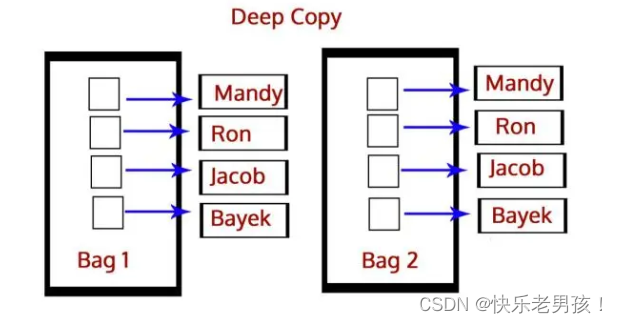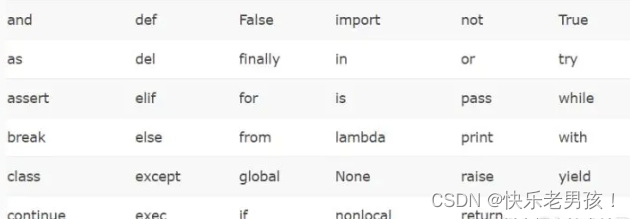### 最常见的Python面试题&答案（上篇）

Python新手在谋求一份Python编程工作前，必须熟知Python的基础知识。编程网站DataFlair的技术团队分享了一份最常见Python面试题合集，既有基本的Python面试题，也有高阶版试题来指导你准备面试，试题均附有答案。面试题内容包括编码、数据结构、脚本撰写等话题。本文为上篇。

• 可解释
• 具有动态特性
• 面向对象
• 简明简单
• 开源
• 具有强大的社区支持

### Q 2：深拷贝和浅拷贝之间的区别是什么？

``>>> import copy>>> b=copy.deepcopy(a)````````>>> b=copy.copy(a)
``````

### Q 3. 列表和元组之间的区别是？

``>>> mylist=[1,3,3]>>> mylist=2>>> mytuple=(1,3,3)>>> mytuple=2Traceback (most recent call last):File "<pyshell#97>", line 1, in <module>mytuple=2``

``````TypeError: ‘tuple’ object does not support item assignment
``````

### Q 4. 解释一下Python中的三元运算子

``````[on true] if [expression] else [on false]
``````

``>>> a,b=2,3>>> min=a if a<b else b>>> min``

``````2
``````
``````>>> print("Hi") if a<b else print("Bye")
``````

``````Hi
``````

### Q 5. 在Python中如何实现多线程？

Python中的GIL（全局解释器锁）确保一次执行单个线程。一个线程保存GIL并在将其传递给下个线程之前执行一些操作，这会让我们产生并行运行的错觉。但实际上，只是线程在CPU上轮流运行。当然，所有的传递会增加程序执行的内存压力。

### Q 6. 解释一下Python中的继承

• 单继承：一个类继承自单个基类
• 多继承：一个类继承自多个基类
• 多级继承：一个类继承自单个基类，后者则继承自另一个基类
• 分层继承：多个类继承自单个基类
• 混合继承：两种或多种类型继承的混合

### Q 8. 在Python中是如何管理内存的？

Python有一个私有堆空间来保存所有的对象和数据结构。作为开发者，我们无法访问它，是解释器在管理它。但是有了核心API后，我们可以访问一些工具。Python内存管理器控制内存分配。

### Q 9. 解释Python中的help()和dir()函数

Help()函数是一个内置函数，用于查看函数或模块用途的详细说明：

``>>> import copy>>> help(copy.copy)``

``Help on function copy in module copy:   copy(x) Shallow copy operation on arbitrary Python objects. See the module’s __doc__ string for more info.``

Dir()函数也是Python内置函数，dir() 函数不带参数时，返回当前范围内的变量、方法和定义的类型列表；带参数时，返回参数的属性、方法列表。

``````>>> dir(copy.copy)
``````

``````[‘__annotations__’, ‘__call__’, ‘__class__’, ‘__closure__’, ‘__code__’, ‘__defaults__’, ‘__delattr__’, ‘__dict__’, ‘__dir__’, ‘__doc__’, ‘__eq__’, ‘__format__’, ‘__ge__’, ‘__get__’, ‘__getattribute__’, ‘__globals__’, ‘__gt__’, ‘__hash__’, ‘__init__’, ‘__init_subclass__’, ‘__kwdefaults__’, ‘__le__’, ‘__lt__’, ‘__module__’, ‘__name__’, ‘__ne__’, ‘__new__’, ‘__qualname__’, ‘__reduce__’, ‘__reduce_ex__’, ‘__repr__’, ‘__setattr__’, ‘__sizeof__’, ‘__str__’, ‘__subclasshook__’]
``````

### Q 11. 什么是猴子补丁？

``>>> class A:    def func(self):        print("Hi")>>> def monkey(self):print "Hi, monkey">>> m.A.func = monkey>>> a = m.A()>>> a.func()``

``````Hi, Monkey
``````

### Q 12. Python中的字典是什么？

``>>> roots={25:5,16:4,9:3,4:2,1:1}>>> type(roots)<class 'dict'>>>> roots``

``````3
``````

``>>> roots={x**2:x for x in range(5,0,-1)}>>> roots``

``````{25: 5, 16: 4, 9: 3, 4: 2, 1: 1}
``````

### Q 13. 请解释使用*args和**kwargs的含义

``>>> def func(*args):    for i in args:        print(i)  >>> func(3,2,1,4,7)``

``3 2 1 4 7``

``>>> def func(**kwargs):    for i in kwargs:        print(i,kwargs[i])>>> func(a=1,b=2,c=7)``

``a.1 b.2 c.7``

### Q 14. 请写一个Python逻辑，计算一个文件中的大写字母数量

``>>> import os >>> os.chdir('C:\\Users\\lifei\\Desktop')>>> with open('Today.txt') as today:    count=0    for i in today.read():        if i.isupper():            count+=1print(count)``

``````26
``````

### Q 15. 什么是负索引？

``````>>> mylist=[0,1,2,3,4,5,6,7,8]
``````

``````>>> mylist[-3]
``````

``````6
``````

``````>>> mylist[-6:-1]
``````

``````[3, 4, 5, 6, 7]
``````

### Q 16. 如何以就地操作方式打乱一个列表的元素？

``>>> from random import shuffle>>> shuffle(mylist)>>> mylist``

``````[3, 4, 8, 0, 5, 7, 6, 2, 1]
``````

### Q 17. 解释Python中的join()和split()函数

Join()能让我们将指定字符添加至字符串中。

``````>>> ','.join('12345')
``````

``````‘1,2,3,4,5’
``````

Split()能让我们用指定字符分割字符串。

``````>>> '1,2,3,4,5'.split(',')
``````

``````[‘1’, ‘2’, ‘3’, ‘4’, ‘5’]
``````

### Q 18. Python区分大小写吗？

``>>> myname='Ayushi'>>> MynameTraceback (most recent call last):File "<pyshell#3>", line 1, in <module>``

``Myname NameError: name ‘Myname’ is not defined``

### Q 19. Python中的标识符长度能有多长？

1. 只能以下划线或者 A-Z/a-z 中的字母开头

2. 其余部分可以使用 A-Z/a-z/0-9

3. 区分大小写

4. 关键字不能作为标识符，Python中共有如下关键字：### Q 20. 怎么移除一个字符串中的前导空格？

``````>>> '   Ayushi '.lstrip()
``````

``````‘Ayushi   ’
``````

``````>>> '   Ayushi '.rstrip()
``````

``````‘   Ayushi’
``````

### Q 21. 怎样将字符串转换为小写？

``````>>> 'AyuShi'.lower()
``````

``````‘ayushi’
``````

``````>>> 'AyuShi'.upper()
``````

``````‘AYUSHI’
``````

``>>> 'AyuShi'.isupper()False >>> 'AYUSHI'.isupper()True >>> 'ayushi'.islower()True >>> '@yu\$hi'.islower()True >>> '@YU\$HI'.isupper()True``

Istitle()能告诉我们一个字符串是否为标题格式。

``>>> 'The Corpse Bride'.istitle()True``

### Q 22. Python中的pass语句是什么？

`` >>> def func(*args):           pass >>>``

``>>> for i in range(7):    if i==3: breakprint(i)``

``0 1 2``

``>>> for i in range(7):    if i==3: continueprint(i)``

``0 1 2 4 5 6``

### Q 23. Python中的闭包是什么？

``>>> def A(x):    def B():        print(x)    return B>>> A(7)()``

``````7
``````

### Q 24. 解释一下Python中的//，%和 ** 运算符

//运算符执行地板除法（向下取整除），它会返回整除结果的整数部分。

``>>> 7//23``

``>>> 2**101024``

``>>> 13%76>>> 3.5%1.50.5``

### Q 25. 在Python中有多少种运算符？解释一下算数运算符。

1.加号（+），将两个值相加

``>>> 7+815``

2.减号（-），将第一个值减去第二个值

``>>> 7-8-1``

3.乘号（*），将两个值相乘

``>>> 7*856``

4.除号（/），用第二个值除以第一个值

``>>> 7/80.875>>> 1/11.0``

5.向下取整除、取模和取幂运算，参见上个问题。

### Q 26. 解释一下Python中的关系运算符

1.小于号（<），如果左边的值较小，则返回True。

``>>> 'hi'<'Hi'False``

2.大于号（>），如果左边的值较大，则返回True。

``>>> 1.1+2.2>3.3True``

3.小于等于号（<=），如果左边的值小于或等于右边的值，则返回Ture。

``>>> 3.0<=3True``

4.大于等于号（>=），如果左边的值大于或等于右边的值，则返回True。

``>>> True>=FalseTrue``
1. 等于号（==），如果符号两边的值相等，则返回True。
``>>> {1,3,2,2}=={1,2,3}True``
1. 不等于号（!=），如果符号两边的值不相等，则返回True。
``>>> True!=0.1True>>> False!=0.1True``

### Q 27. 解释一下Python中的赋值运算符

``>>> a=7>>> a+=1>>> a8 >>> a-=1>>> a7 >>> a*=2>>> a14 >>> a/=2>>> a7.0  >>> a**=2>>> a49 >>> a//=3>>> a16.0 >>> a%=4>>> a0.0``

### Q 28. 解释一下Python中的逻辑运算符

Python中有3个逻辑运算符：and，or，not。

``>>> False and TrueFalse >>> 7<7 or TrueTrue >>> not 2==2False``

### Q 29. 解释一下Python中的成员运算符

``>>> 'me' in 'disappointment'True >>> 'us' not in 'disappointment'True``

### Q 30. 解释一下Python中的身份运算符

``>>> 10 is '10'False >>> True is not FalseTrue``

### Q 31. 讲讲Python中的位运算符

1. 与（&），按位与运算符：参与运算的两个值,如果两个相应位都为1,则该位的结果为1,否则为0
``>>> 0b110 & 0b0102``

2.或（|），按位或运算符：只要对应的二个二进位有一个为1时，结果位就为1。

``>>> 3|23``

3.异或（^），按位异或运算符：当两对应的二进位相异时，结果为1

``>>> 3^21``

4.取反（~），按位取反运算符：对数据的每个二进制位取反,即把1变为0,把0变为1

``>>> ~2-3``

5.左位移（<<），运算数的各二进位全部左移若干位，由 << 右边的数字指定了移动的位数，高位丢弃，低位补0

``>>> 1<<24``

6.右位移（>>），把”>>”左边的运算数的各二进位全部右移若干位，>> 右边的数字指定了移动的位数

``>>> 4>>21``

### Q 32. 在Python中如何使用多进制数字？

1. 二进制数字由0和1组成，我们使用 0b 或 0B 前缀表示二进制数。
``>>> int(0b1010)10``

2.使用bin()函数将一个数字转换为它的二进制形式。

``>>> bin(0xf)‘0b1111’``

3.八进制数由数字 0-7 组成，用前缀 0o 或 0O 表示 8 进制数。

``>>> oct(8)‘0o10’``

4.十六进数由数字 0-15 组成，用前缀 0x 或者 0X 表示 16 进制数。

``>>> hex(16)‘0x10’ >>> hex(15)‘0xf’``

### Q 33. 怎样获取字典中所有键的列表？

``>>> mydict={'a':1,'b':2,'c':3,'e':5}>>> mydict.keys()dict_keys(['a', 'b', 'c', 'e'])``

### Q 35. 怎样声明多个变量并赋值？

``>>> a,b,c=3,4,5 #This assigns 3, 4, and 5 to a, b, and c respectively>>> a=b=c=3 #This assigns 3 to a, b, and c``

### Q 36. 元组的解封装是什么？

``>>> mytuple=3,4,5>>> mytuple(3, 4, 5)``

``>>> x,y,z=mytuple>>> x+y+z``

### 结语